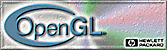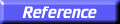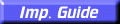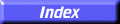# glCopyPixels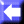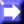glCopyPixels: copy pixels in the frame buffer.

C Specification | Parameters | Description | Examples | Notes | Errors | Associated Gets | See Also

##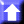C Specification

```void glCopyPixels(
GLint	 x,
GLint	 y,
GLsizei	 width,
GLsizei	 height,
GLenum	 type)
```

##Parameters

x, y
Specify the window coordinates of the lower left corner of the rectangular region of pixels to be copied.
width, height
Specify the dimensions of the rectangular region of pixels to be copied. Both must be nonnegative.
type
Specifies whether color values, depth values, or stencil values are to be copied. Symbolic constants GL_COLOR, GL_DEPTH, and GL_STENCIL are accepted.

##Description

glCopyPixels copies a screen-aligned rectangle of pixels from the specified frame buffer location to a region relative to the current raster position. Its operation is well defined only if the entire pixel source region is within the exposed portion of the window. Results of copies from outside the window, or from regions of the window that are not exposed, are hardware dependent and undefined.

x and y specify the window coordinates of the lower left corner of the rectangular region to be copied. width and height specify the dimensions of the rectangular region to be copied. Both width and height must not be negative.

Several parameters control the processing of the pixel data while it is being copied. These parameters are set with three commands: glPixelTransfer, glPixelMap, and glPixelZoom. This reference page describes the effects on glCopyPixels of most, but not all, of the parameters specified by these three commands.

glCopyPixels copies values from each pixel with the lower left-hand corner at (x + i, y + j) for 0
i<width and 0j<height. This pixel is said to be the ith pixel in the jth row. Pixels are copied in row order from the lowest to the highest row, left to right in each row.

type specifies whether color, depth, or stencil data is to be copied. The details of the transfer for each data type are as follows:

GL_COLOR
Indices or RGBA colors are read from the buffer currently specified as the read source buffer (see glReadBuffer). If the GL is in color index mode, each index that is read from this buffer is converted to a fixed-point format with an unspecified number of bits to the right of the binary point. Each index is then shifted left by GL_INDEX_SHIFT bits, and added to GL_INDEX_OFFSET. If GL_INDEX_SHIFT is negative, the shift is to the right. In either case, zero bits fill otherwise unspecified bit locations in the result. If GL_MAP_COLOR is true, the index is replaced with the value that it references in lookup table GL_PIXEL_MAP_I_TO_I. Whether the lookup replacement of the index is done or not, the integer part of the index is then ANDed with 2b
1, where b is the number of bits in a color index buffer.

If the GL is in RGBA mode, the red, green, blue, and alpha components of each pixel that is read are converted to an internal floating-point format with unspecified precision. The conversion maps the largest representable component value to 1.0, and component value 0 to 0.0. The resulting floating-point color values are then multiplied by GL_c_SCALE and added to GL_c_BIAS, where c is RED, GREEN, BLUE, and ALPHA for the respective color components. The results are clamped to the range [0, 1]. If GL_MAP_COLOR is true, each color component is scaled by the size of lookup table GL_PIXEL_MAP_c_TO_c, then replaced by the value that it references in that table. c is R, G, B, or A.

The GL then converts the resulting indices or RGBA colors to fragments by attaching the current raster position z coordinate and texture coordinates to each pixel, then assigning window coordinates (xr + i , yr + j), where (xr, yr) is the current raster position, and the pixel was the ith pixel in the jth row. These pixel fragments are then treated just like the fragments generated by rasterizing points, lines, or polygons. Texture mapping, fog, and all the fragment operations are applied before the fragments are written to the frame buffer.

GL_DEPTH
Depth values are read from the depth buffer and converted directly to an internal floating-point format with unspecified precision. The resulting floating-point depth value is then multiplied by GL_DEPTH_SCALE and added to GL_DEPTH_BIAS. The result is clamped to the range [0, 1].

The GL then converts the resulting depth components to fragments by attaching the current raster position color or color index and texture coordinates to each pixel, then assigning window coordinates (xr + i, yr + j), where (xr, yr) is the current raster position, and the pixel was the ith pixel in the jth row. These pixel fragments are then treated just like the fragments generated by rasterizing points, lines, or polygons. Texture mapping, fog, and all the fragment operations are applied before the fragments are written to the frame buffer.

GL_STENCIL
Stencil indices are read from the stencil buffer and converted to an internal fixed-point format with an unspecified number of bits to the right of the binary point. Each fixed-point index is then shifted left by GL_INDEX_SHIFT bits, and added to GL_INDEX_OFFSET. If GL_INDEX_SHIFT is negative, the shift is to the right. In either case, zero bits fill otherwise unspecified bit locations in the result. If GL_MAP_STENCIL is true, the index is replaced with the value that it references in lookup table GL_PIXEL_MAP_S_TO_S. Whether the lookup replacement of the index is done or not, the integer part of the index is then ANDed with 2b1, where b is the number of bits in the stencil buffer. The resulting stencil indices are then written to the stencil buffer such that the index read from the ith location of the jth row is written to location (xr + i, yr + j), where (xr, yr) is the current raster position. Only the pixel ownership test, the scissor test, and the stencil writemask affect these write operations.
The rasterization described thus far assumes pixel zoom factors of 1.0. If glPixelZoom is used to change the x and y pixel zoom factors, pixels are converted to fragments as follows. If (xr, yr) is the current raster position, and a given pixel is in the ith location in the jth row of the source pixel rectangle, then fragments are generated for pixels whose centers are in the rectangle with corners at

(xr + zoomxi, yr + zoomy j )
and
(xr + zoomx(i+1), yr + zoomy ( j+1))

where zoomx is the value of GL_ZOOM_X and zoomy is the value of GL_ZOOM_Y.

##Examples

To copy the color pixel in the lower left corner of the window to the current raster position, use glCopyPixels(0, 0, 1, 1, GL_COLOR);

##Notes

Modes specified by glPixelStore have no effect on the operation of glCopyPixels.

##Errors

• GL_INVALID_ENUM is generated if type is not an accepted value.
• GL_INVALID_VALUE is generated if either width or height is negative.
• GL_INVALID_OPERATION is generated if type is GL_DEPTH and there is no depth buffer.
• GL_INVALID_OPERATION is generated if type is GL_STENCIL and there is no stencil buffer.
• GL_INVALID_OPERATION is generated if glCopyPixels is executed between the execution of glBegin and the corresponding execution of glEnd.

##Associated Gets

glGet with argument GL_CURRENT_RASTER_POSITION
glGet with argument GL_CURRENT_RASTER_POSITION_VALID

glDepthFunc,
glDrawBuffer,
glDrawPixels,
glPixelMap,
glPixelTransfer,
glPixelZoom,
glRasterPos,